×

Search anything:

# LSTM & BERT models for Natural Language Processing (NLP)

#### Machine Learning (ML) Natural Language Processing (NLP)Get this book -> Problems on Array: For Interviews and Competitive Programming

There are various NLP models that are used to solve the problem of language translation. In this article, we are going to learn how the basic language model was made and then move on to the advance version of language model that is more robust and reliable.

The fundamental NLP model that is used initially is LSTM model but because of its drawbacks BERT became the favoured model for the NLP tasks.

# LSTM Model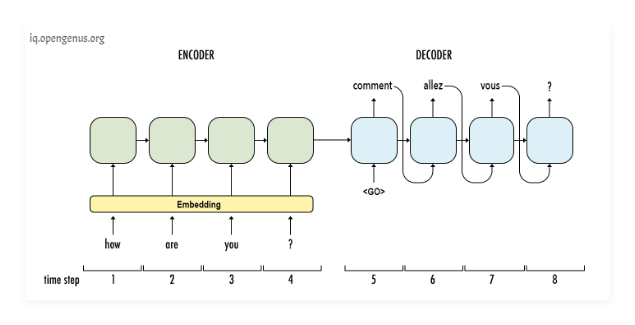Initially LSTM networks had been used to solve the Natural Language Translation problem but they had a few problems. LSTM networks are-

• Slow to train. Words are passed in sequentially and are generated sequentially it can take a significant number of timesteps for the neural net to learn.
• It's not really the best of capturing the true meaning of words, even bi-directional LSTMS are not. Because even here they are technically learning left to right and right to left context separately and then concatenating them so the true context is lost.

# Transformer Architecture

This is the transformer neural network architecture that was initially created to solve the problem of language translation.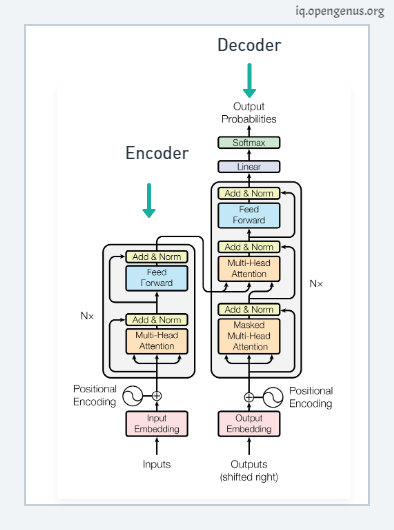Transformer architecture addresses some of the drawback of LSTM:-

• They are faster as words can be processed simultaneously.
• The context of words is better learned as they can learn context from both directions simultaneously.

# Transformer Flow

Now let's see the transformer in action. Say we want to train this architecture to convert English to French.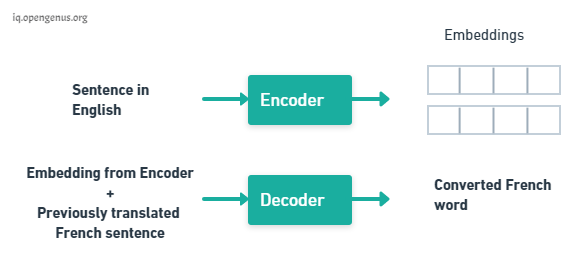The transformer consists of two key components an Encoder and a Decoder.
The Encoder takes the English words simultaneously and it generates embeddings for every word simultaneously these embeddings are vectors that encapsulate the meaning of the word, similar words have closer numbers in their vectors. The Decoder takes these embeddings from the Encoder and the previously generated words of the translated French sentence and then it uses them to generate the next French word and we keep generating the French translation one word at a time until the end of sentence is reached.

What makes Transformer conceptually stronger than LSTM cell is that we can physically see a separation in tasks. The Encoder learns What is English and its grammar? and What is context? The Decoder learns how do English words relate to French words. Separately they both have some underlying understanding of language and it's because of this understanding that we can pick apart this architecture and build systems that understand language.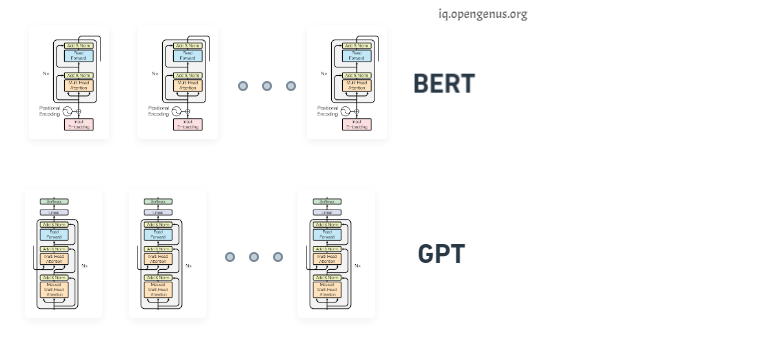We stack the decoders and we get the GPT (Generative Pre-training) transformer architecture, conversely if we stack just the encoders we get BERT a bi-directional encoder representation from transformer.

# BERT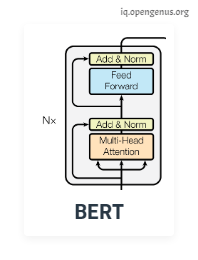We can use BERT for problems which needs Language understanding:

• Neural Machine Translation
• Sentiment Analysis
• Text summarization

These problems can be solved by BERT Training phases which are:

1. Pretain BERT to understand language and context.
2. Fine tune BERT to learn how to solve a specific task.

# 1. Pre-training

The goal of pre training is to make BERT learn what is language and what is context? BERT learns language by training on two Unsupervised tasks simultaneously, they are Mass Language Modeling (MLM) and Next Sentence Prediction (NSP).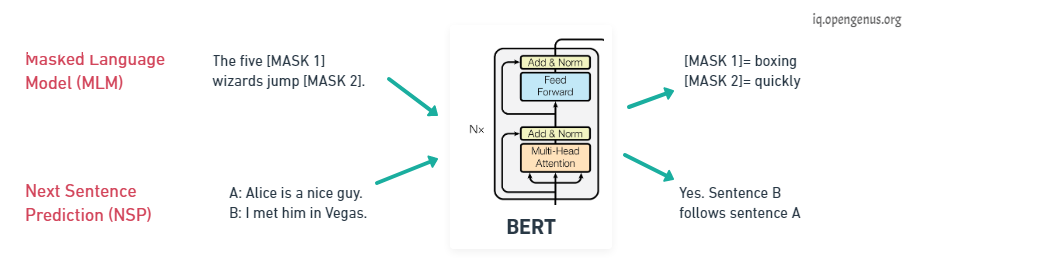For Mass Language Modeling, BERT takes in a sentence with random words filled with masks. The goal is to output these masked tokens and this is kind of like fill in the blanks it helps BERT understand a bi-directional context within a sentence.

In the case of Next Sentence Prediction, BERT takes in two sentences and it determines if the second sentence actually follows the first, in kind of like a binary classification problem. This helps BERT understand context across different sentences themselves and using both of these together BERT gets a good understanding of language.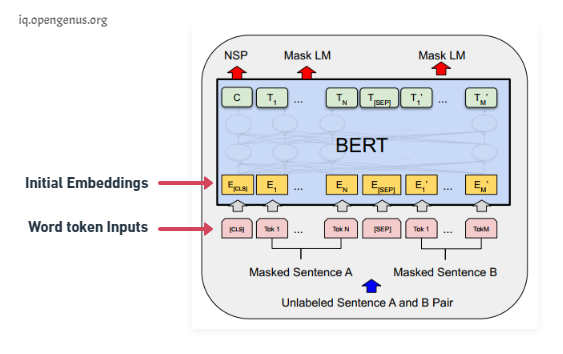During BERT pre-training the training is done on Mass Language Modeling and Next Sentence Prediction. In practice both of these problems are trained simultaneously, the input is a set of two sentences with some of the words being masked (each token is a word) and convert each of these words into embeddings using pre-trained embeddings. On the output side C is the binary output for the next sentence prediction so it would output 1 if sentence B follows sentence A in context and 0 if sentence B doesn't follow sentence A. Each of the T's here are word vectors that correspond to the outputs for the mass language model problem, so the number of word vectors that is input is the same as the number of word vectors that we got as output.

On the input side, how are we going to generate embeddings from the word token inputs?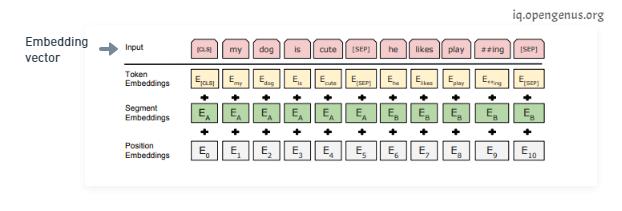The initial embedding is constructed from three vectors, the token embeddings are the pre-trained embeddings; the main paper uses word-pieces embeddings that have a vocabulary of 30,000 tokens. The segment embeddings is basically the sentence number that is encoded into a vector and the position embeddings is the position of a word within that sentence that is encoded into a vector. Adding these three vectors together we get an embedding vector that we use as input to BERT. The segment and position embeddings are required for temporal ordering since all these vectors are fed in simultaneously into BERT and language models need this ordering preserved.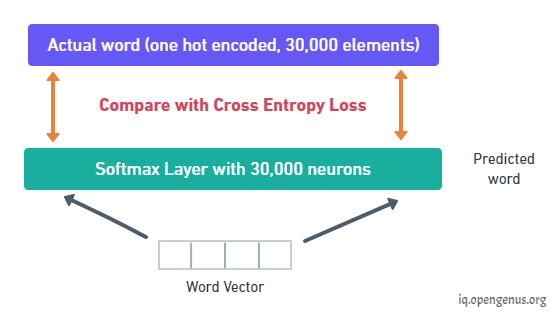The output is a binary value C and a bunch of word vectors but with training we need to minimize a loss. So two key details to note here all of these word vectors have the same size and all of these word vectors are generated simultaneously, we need to take each word vector pass it into a fully connected layered output with the same number of neurons equal to the number of tokens in the vocabulary so that would be an output layer corresponding to 30,000 neurons in this case and we would apply a softmax activation. This way we would convert a word vector to a distribution and the actual label of this distribution would be a one hot encoded vector for the actual word and so we compare these two distributions and then train the network using the cross entropy loss.

But note that the output has all the words even though those inputs weren't masked at all. The loss though only considers the prediction of the masked words and it ignores all the other words that are output by the network this is done to ensure that more focus is given to predicting [MASK]ed values so that it gets them correct and it increases context awareness.

Once training is complete BERT has some notion of language as it's a language model.

# 2. Fine-tuning

We can now further train BERT on very specific NLP tasks for example let's take question answering, all we need to do is replace the fully connected output layers of the network with a fresh set of output layers that can basically output the answer to the question we want.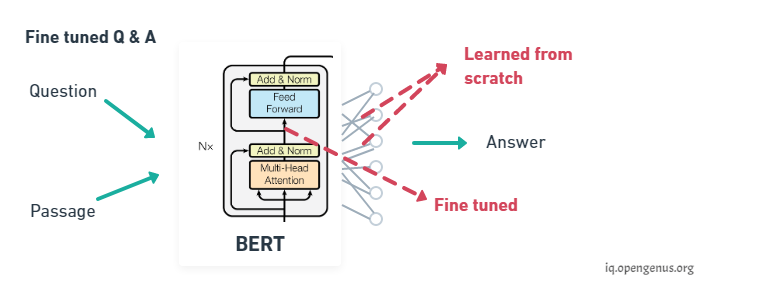Then supervised training can be performed using a question answering dataset it won't take long since it's only the output parameters that are learned from scratch, the rest of the model parameters are just slightly fine-tuned and as a result training time is fast. This can be done for any NLP problem that is replace the output layers and then train with a specific dataset.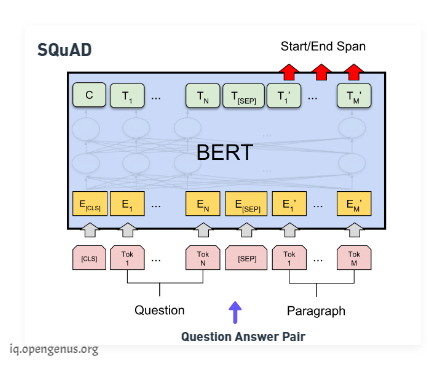Now on the fine tuning phase, if we wanted to perform question-answering we would train the model by modifying the inputs and the output layer. We pass in the question followed by a passage containing the answer as inputs and in the output layer we would output Start and the End words that encapsulate the answer assuming that the answer is within the same span of text.

BERT SQuAD that is the Stanford Question-and-answer Dataset only takes about 30 minutes to fine-tune from a language model for a 91% performance.

Of course performance depends on how big we want BERT to be. The BERT large model which has 340 million parameters can achieve way higher accuracies than the BERT base model which only has 110 parameters.

References
 BERT main paper: https://arxiv.org/pdf/1810.04805.pdf
 Overview of BERT: https://arxiv.org/pdf/2002.12327v1.pdf